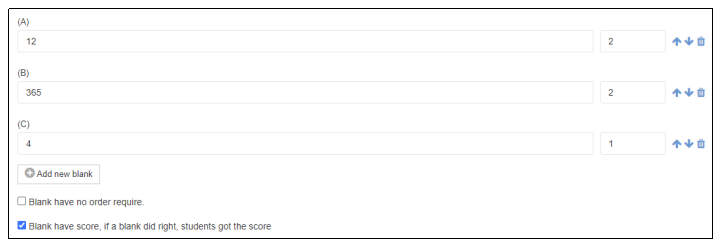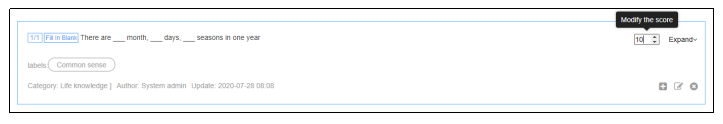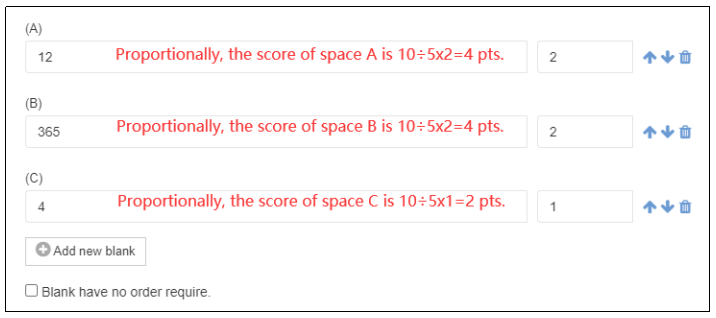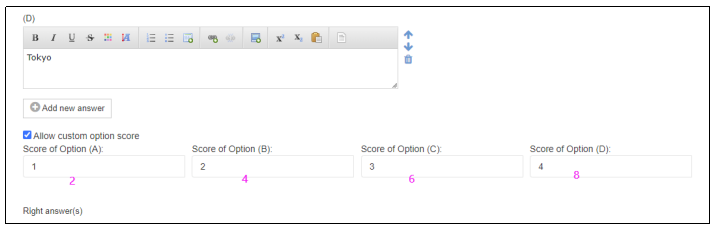# Exams Online Testing Platform - Online Exam Platform

## Instructions for Modifying Test Scores

If the user adds test questions when composing a test paper (or when editing test questions in [Questions Bank]) , in principle, the system defaults the score of each question to the score set when importing, but the user can modify the score of each question. If you need to modify the test scores in a test paper, please read the following instructions carefully:

1. The test questions imported into the system can be used in multiple test papers. If the score of a test question is modified when composing a paper, only the score of the question in the current test paper is modified. It will only be used once, and will not affect the scores of other test papers. The score displayed in the question bank will not change either.

2. If you need to modify the score of a test question in a test paper, the test score will follow the following rules:

(1) After the test score is modified, the score that candidates get when they answer the question correctly will be based on the modified score, not the score when the test question is imported.

For example, if a question is 2 points, but the score is changed to 10 when the test question is added to the test paper, then the candidates will get a score of 10.(2) If the fill-in-the-blank questions are set to be scored as blanks during import, after the scores are modified, the system will allocate the scores for each blank according to the proportion.

For example, a fill-in-the-blank question with three spaces, when originally imported, the scores for each space are 2, 2, and 1 (total score is 5);When adding the test question to the test paper, change the total score of test question from 5 to 10, then each space is calculated in proportion, and the score will be 4, 4, and 2.(3) When multiple choice questions are set with custom option scores, after modifying the scores, the system will allocate the scores of each option according to the proportion.

For example, the scores of the option ABCD of a multiple-choice question are 1, 2, 3, 4 (total score is 10), and the modified score is 20. The scores of the four options are calculated as 2, 4, 6, and 8.